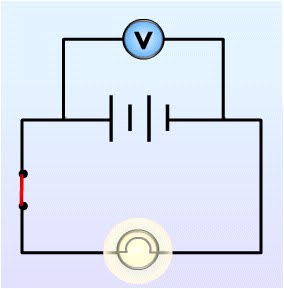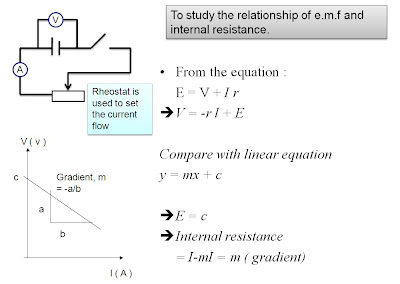## Monday, March 8, 2010

### Electric powerWhen an electrical appliance is switched on, the current flows and the electrical energy supplied by the source is transformed to other forms of energy (e.g heat and light energy in electric bulb)
Therefore, we can define electrical energy as :
The energy carried by electrical charges which can be transformed to other forms of energy by the operation of an electrical appliance.

Electric power: rate of transferring electric energy

P = E /t

where P = power [Watt]
E = electric energy used / dissipated [Joule]
t = time [seconds]

P = IV

where P = power [Watt]
I = current [Ampere]
V = potential difference [Volt]

From P = IV and V = IR,
P = I2 R
P = V2 /RElectrical appliances are usually labeled by its voltage and power rating
Voltage – required potential difference to operate the appliance
Power rating – energy dissipated by the appliance when the correct voltage is supplied

## Saturday, March 6, 2010

### Internal resistanceBefore the switch is turned on
• The battery does not supply current to the light bulb
• Voltmeter reading = E.m.f. of batteryAfter the switch is turned on
• The battery supplies current which flows around the circuit
• Voltmeter measures the potential difference across the terminals of the battery
• The voltmeter reading drops due to internal resistance of the batteryEMF = IR + Ir
EMF = V + Ir
EMF = I (R + r)

where EMF = electromotive force [V]
I = current flowing through the circuit [A]
R = total resistance of the circuit [Ω]
r = internal resistance of the batteries [Ω]
V = potential difference of the circuit [V]Worked example:

What is the internal resistance of a battery if its emf is 12 V and the voltage drop across its terminals is 10 V when a current of 4 A ﬂows in the circuit when it is connected across a load?

Solution:
E = V + Ir

12 = 10 + 4(r)

r =0.5

## Thursday, March 4, 2010

### Electromotive Force , E.M.FWhen you measure the potential diﬀerence across (or between) the terminals of a battery you are measuring the ”electromotive force” (emf) of the battery.
This is how much potential energy the battery has to make charges move through the circuit. This driving potential energy is equal to the total potential energy drops in the circuit. This means that the voltage across the battery is equal to the sum of the voltages in the circuit.

E.m.f. = sum of potential difference across the whole circuit, Vtotal

Worked example :What is the voltage across the unknown resistor in the circuit shown?

Solution :
Vbattery = Vtotal

Vbattery = V1 + Vresistor

3V = V1 + 1V

V1 = 2V

## Tuesday, March 2, 2010

### Parallel CircuitThe potential difference is the same across each resistor
V = V1 = V2

The current passing through each resistor is inversely proportional to the resistance of the resistor. The current in the circuit equals to the sum of the currents passing through the
resistors in its parallel branches.

I = I1 + I2

where I is the total current from the battery

If Ohm’s law is applied separately to each resistor, we get :
I = I1 + I2

V/R = V/R1 + V/R2

If each term in the equation is divided by V, we get the effective resistance

1/R = 1/R1 + 1/R2

Worked example :The electrical current flowing through each branch, I1 and I2, is 5 A. Both bulbs have the same resistance, which is 2Ω. Calculate the voltage supplied.

solution:
Parallel circuit;V =V1=V2 = IR1 or = IR2 = 5(2) = 10 V

## Monday, March 1, 2010

### PEKA form 5 ( p.d and current)

5 um and 5 ukm students please refer to the peka format done by Miss Ada from 5 um .